### Compton Scattering

Compton scattered high energy photons from (essentially) free electrons in 1923. He measured the wavelength of the scattered photons as a function of the scattering angle. The figure below shows both the initial state (a) and the final state, with the photon scattered by an angle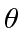and the electron recoiling at an angle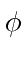. The photons were from nuclear decay and so they were of high enough energy that it didn't matter that the electrons were actually bound in atoms. We wish to derive the formula for the wavelength of the scattered photon as a function of angle.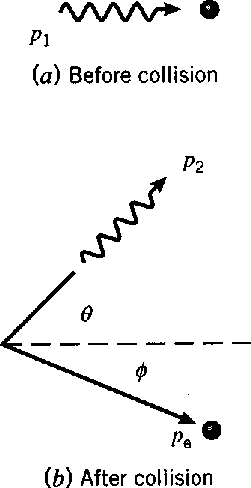With two final state particles, we have 6 unknowns and 4 conservation equations, leaving two variables undetermined. One of these is the uninteresting azimuthal angle (rotation about the beam direction). The other is the scattering angle for the photonwhich is not determined but interesting. The probability distribution inmay tell us something about the interaction responsible for the scattering but our analysis is only of the kinematics. We will simply calculate what the energy is for the scattered photon as a function of the scattering angle.

We solve the problem using only conservation of energy and momentum. Lets work in units in which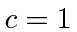for now. We'll put theback in at the end. Assume the photon is initially moving in thedirection with energy E and that it scatters in theplane so that. Conservation of momentum gives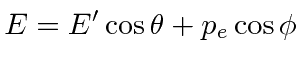andConservation of energy gives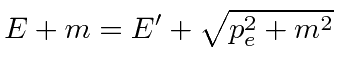Our goal is to solve forin terms of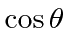so lets make sure we eliminate the. Continuing from the energy equation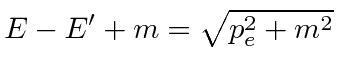squaring and calculatingfrom the components we eliminateand writing out the squares on the right sideand removing things that appear on both sidesand grouping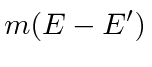Sincein our fine units,We now apply the speed of light to make the units come out to be a length.These calculations can be fairly frustrating if you don't decide which variables you want to keep and which you need to eliminate from your equations. In this case we eliminatedby using the energy equation and computing.

Jim Branson 2012-10-21